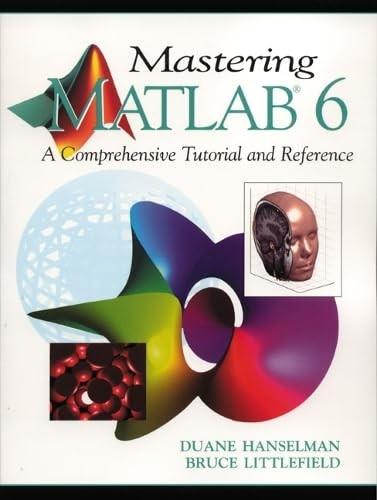Free Shipping in Australia
Proud to be B-Corp

# Mastering MATLAB 6 Duane Hanselman\$36.99
Condition - Very Good
Only 2 left

## Summary

Suitable for undergraduate and graduate courses in MATLAB, or as a reference wherever MATLAB is used.

This text covers all essential aspects of MATLAB presented within an easy-to-follow learn while doing tutorial format.

## Mastering MATLAB 6 Summary

### Mastering MATLAB 6: United States Edition by Duane Hanselman

Suitable for undergraduate courses in MATLAB, or as a reference for any course where MATLAB is used. This comprehensive text covers all aspects of MATLAB presented within an easy-to-follow learn while doing tutorial format. *NEW - All new features of MATLAB version 6 covered. *Demonstrates how the new features can be used to increase productivity and do new things. *NEW - Integration of MATLAB with C, FORTRAN, and Java covered. *Increases MATLABs power and flexibility in dealing with external algorithms, datasets, and operating system capabilities. *NEW - More thorough coverage of indexing, vectorizing, and linear algebra. *Increases ones problem solving productivity and dramatically increases algorithm speed. *NEW - More use of examples - Every chapter offers more extensive examples than the previous editions of Mastering MATLAB. In addition, a chapter specifically covering extensive examples is included. *NEW - A more comprehensive index - The index is extensively revised and expanded.

1. Getting Started.

2. Basic Features.

3. The MATLAB Desktop.

4. Script M-Files.

5. Arrays and Array Operations.

6. Multidimensional Arrays.

7. Cell Arrays and Structures.

8. Character Strings.

9. Relational and Logical Operations.

10. Control Flow.

11. Function M-Files.

12. M-File Debugging and Profiling.

13. File and Directory Management.

14. Set, Bit, and Base Functions.

15. Time Computations.

16. Matrix Algebra.

17. Data Analysis.

18. Data Interpolation.

19. Polynomials.

20. Cubic Splines.

21. Fourier Analysis.

22. Optimization.

23. Integration and Differentiation.

24. Differential Equations.

25. Two-Dimensional Graphics.

26. Three-Dimensional Graphics.

27. Using Color and Light.

28. Images, Movies, and Sound.

29. Printing and Exporting Graphics.

30. Handle Graphics.

31. Graphical User Interfaces.

32. Dialog Boxes.

33. MATLAB Classes and Object-Oriented Programming.

34. MATLAB Programming Interfaces.

35. Extending MATLAB with Java.

36. Windows Application Integration.

37. Getting Help.

38. Examples, Examples, Examples.

Appendices.

Appendix A: Common Handle Graphics Properties.

Appendix B: Axes Object Properties.

Appendix C: Figure Object Properties.

Appendix D: Image Object Properties.

Appendix E: Light Object Properties.

Appendix F: Line Object Properties.

Appendix G: Patch Object Properties.

Appendix H: Rectangle Object Properties.

Appendix I: Root Object Properties.

Appendix J: Surface Object Properties.

Appendix K: Text Object Properties.

Appendix M: Uicontrol Object Properties.

Index.

GOR002042825
9780130194688
0130194689
Mastering MATLAB 6: United States Edition by Duane Hanselman
Used - Very Good
Paperback
Pearson Education (US)
20010103
832
N/A
Book picture is for illustrative purposes only, actual binding, cover or edition may vary.
This is a used book - there is no escaping the fact it has been read by someone else and it will show signs of wear and previous use. Overall we expect it to be in very good condition, but if you are not entirely satisfied please get in touch with us